# How to Convert Multiple Rows into a Single Row in Excel

This post will guide you how to convert multiple rows or columns into a single row with a formula in Excel 3013/2016. How do I convert groups of rows to a single row of columns with VBA Macro in Excel.

## Convert Multiple Row into Single Row with Formula

Assuming that you have a list of data in range A1:B6, and you want to convert those data into a single row in your worksheet, how to do it. You can use a formula based on the OFFSET function, the ROW function, the FLOOR function and the COLUMN function to achieve the result. Like this:

`=OFFSET(Sheet8!\$A\$1,((ROW()-1)*6)+(FLOOR(COLUMN()-1,2)/2),(COLUMN()-1)-(FLOOR(COLUMN()-1,2)))`

You need to type this formula into the cell A1 in a new worksheet in your current workbook. And then drag the AutoFill handle to right until you get the number 0.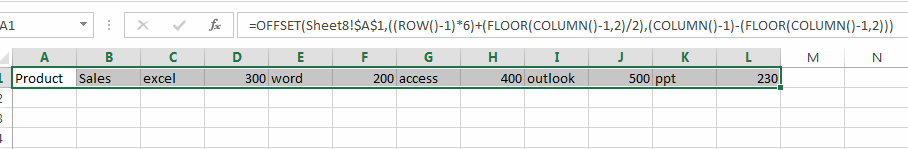Note: the number 6 is the total number or rows. and the number 2 is the total number of  columns.

## Convert Multiple Row into Single Row with VBA Macro

You can also use an Excel VBA Macro to achieve the same result of converting multiple rows or columns into a specified row. Here are the steps:

#1 open your excel workbook and then click on “Visual Basic” command under DEVELOPER Tab, or just press “ALT+F11” shortcut.#2 then the “Visual Basic Editor” window will appear.

#3 click “Insert” ->”Module” to create a new module.#4 paste the below VBA code  into the code window. Then clicking “Save” button.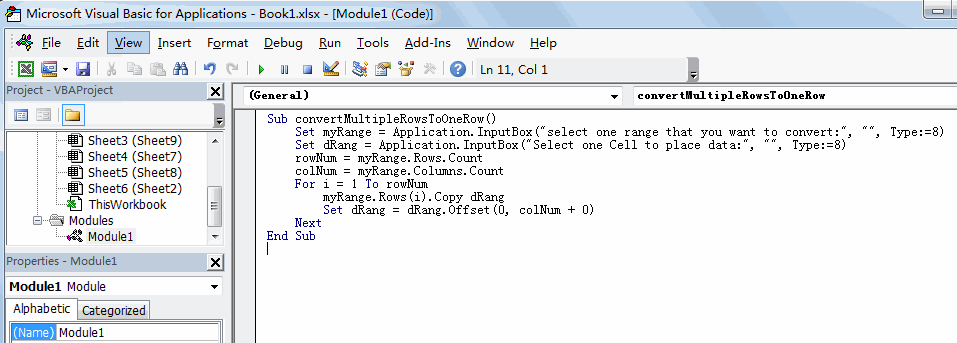```Sub convertMultipleRowsToOneRow()
Set myRange = Application.InputBox("select one range that you want to convert:", "", Type:=8)
Set dRang = Application.InputBox("Select one Cell to place data:", "", Type:=8)
rowNum = myRange.Rows.Count
colNum = myRange.Columns.Count
For i = 1 To rowNum
myRange.Rows(i).Copy dRang
Set dRang = dRang.Offset(0, colNum + 0)
Next
End Sub```

#5 back to the current worksheet, then run the above excel macro. Click Run button.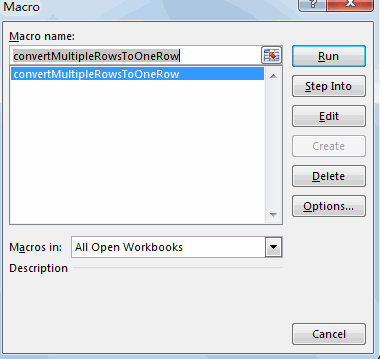#6 select one range that you want to convert. Click OK button.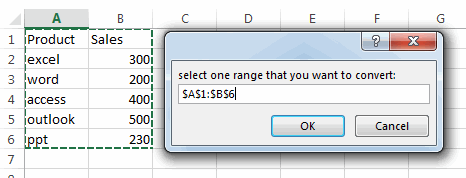#7 Select one Cell to place data.  Click OK button.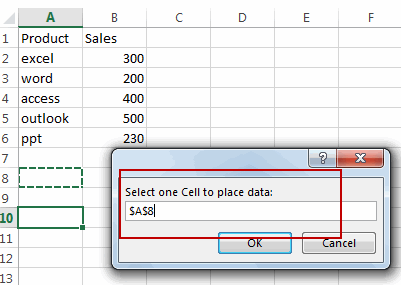#8 Let’s see the result: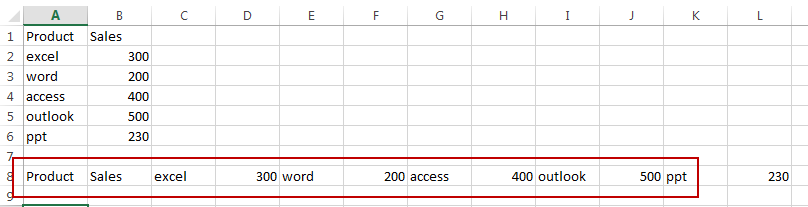### Related Functions

• Excel COLUMN function
The Excel COLUMN function returns the first column number of the given cell reference.The syntax of the COLUMN function is as below:=COLUMN ([reference])….
• Excel ROW function
The Excel ROW function returns the row number of a cell reference.The ROW function is a build-in function in Microsoft Excel and it is categorized as a Lookup and Reference Function.The syntax of the ROW function is as below:= ROW ([reference])….
• Excel FLOOR function
The Excel FLOOR function returns a number rounded down to the nearest multiple of significance. So it will return a rounded number.The syntax of the FLOOR function is as below:= FLOOR (number, significance)…